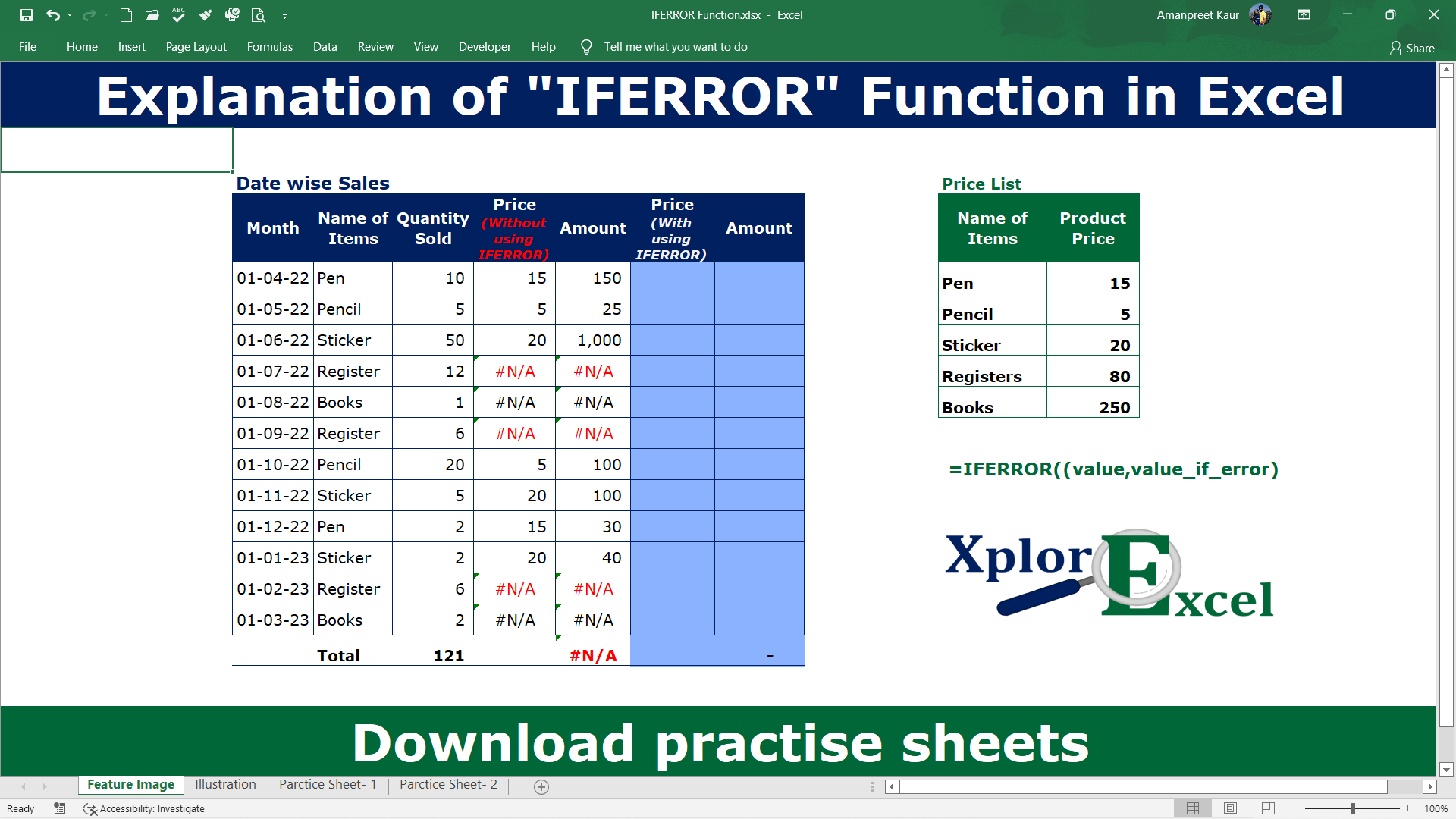# IFERROR Excel Function- Free 2 Practice workbooksExplanation of IFERROR Function in Excel-min

In this article, I have explained to you, how to use the IFERROR Excel Function in Excel with other Excel Formulas and Functions Like, IFERROR with VLOOKUP, IFERROR with HLOOKUP, IFERROR with Simple Divide Formula, and IFERROR with IF. So let’s start.

## IFERROR Excel Function

The IFERROR Excel Function can use with other excel functions. The IFERROR function shows the already given sentence when there is an error in the result of other functions or formulas of excel. Error in result such as #N/A, #VALUE!, #REF!, #DIV/0!, #NUM!, #NAME? or #NULL.

We can use this function where we know that the function will generate errors in some cases. Suppose, if you have created the formula of dividing two cell values with each other but for the time being, these cells don’t have any value. Then The result will show an error of #DIV/0!. So if you use the formula with the IFERROR function then you can remove this result with your predefined text or blank. Now question is that how? In this article, I will explain to you how you can use IFERROR Function with other excel Formulas and Functions.

### The feature of the “IFERROR” function

• IFERROR will find results until it got an error.
• With the help of the IFERROR Function, we can make our project neet and clear by replacing errors with predefined text or Blank Cell.
• It remove/Replaced excel errors only which are logical errors not human errors.
• It can be used with all other fucntions of excel.
• Errors evaluated by IFERROR Function are N/A, #VALUE!, #REF!, #DIV/0!, #NUM!, #NAME? or #NULL.

## Explanation of IFERROR Function

Now, We will explain the Arguments of the Function.

=IFERROR(value, value_if_error)

value:- Select the value on which you want to apply the error rule. You can select the cell or write the already applied formula here.

value_if_error: – You have to write that value which you want to show if there is an error in the applied function. it can be yes, no, 1,0, blank, etc. whatever you want to show.

Following errors are evaluated by this formula:
#N/A, #VALUE!, #REF!, #DIV/0!, #NUM!, #NAME? or #NULL.

## Example of Explanation of IFERROR Function: –

In the following table, we want to retrieve the result of the price of the product without any error message. The table of date-wise sales given in the workbook.

### Solution:

We will Apply the IFERROR function and get the result. This is shown in the following steps: –

#### Step No. 1:-

We will write the “=IFERROR( ” in the column of the result

#### Step No. 2

Now select the value(function) from which you want to check the errors. Like I am coping already applied formula of VLOOKUP in column E and paste it in the value part of IFERROR Function.

you can also write the new formula or any formula for calculation that you are using while calculating something.

#### Step No. 3: –

Now please select the value_if_error that will be shown when there is an error in the selected function.

I have just written 0 because I want the value of 0 only. You can write any message here but if you want to use text then use it within inverted commas like “your text”.

#### Step No. 4: –

Now, copy the newly created formula from cell G4 and paste it into the remaining cell to check out the error from the all given data.

The result of this activity is shown as under:

#### Step No. 5

Now you can multiply the quantity with the new price which got with the IFERROR. Then you will get the amount in column H4.